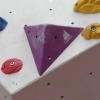#### You may also likeA quadrilateral changes shape with the edge lengths constant. Show the scalar product of the diagonals is constant. If the diagonals are perpendicular in one position are they always perpendicular?### Three by One

There are many different methods to solve this geometrical problem - how many can you find?### Tetra Perp

Show that the edges AD and BC of a tetrahedron ABCD are mutually perpendicular when: AB²+CD² = AC²+BD².

# Quaternions and Rotations

##### Age 16 to 18 Challenge Level:
Comparing the results in parts (1) and (2) we see that in part (1) $q = \cos 45^o + \sin 45^o{\bf i}$ and the map $qvq^{-1}$ fixes the x-axis and gives a rotation of twice 45 degrees about the x-axis.

The result in (2) is slightly more general showing that where $q = \cos \theta + \sin \theta {\bf k}$ the map $q v q^{-1}$ fixes the z-axis and gives a rotation of $2\theta$ about the z-axis. \par For a more general account of quaternions and rotations see the article....